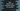# How to find the ceil and floor values of a number in Dart## How to find the ceil and floor values of a number in Dart:

ceil of a number is the smallest integer value that is greater than or equal to that number. Similarly, floor of a number is the largest number that is less than or equal to that number.

To find the ceil and floor, Number class of dart provides two methods called Number.ceil() and Number.floor() that we can use.

### Syntax of floor and ceil functions:

ceil and floor functions are defined as below:

``````Number.ceil()

Number.floor()``````

### Example of floor and ceil:

Below example shows how to use floor and ceil :

``````import 'dart:io';

void main() {
print("Enter a number : ");

print("Ceil : \${num.ceil()}, Floor : \${num.floor()}");
}``````

It will print outputs like below:

``````Enter a number :
2.9
Ceil : 3, Floor : 2

Enter a number :
1.2
Ceil : 2, Floor : 1``````